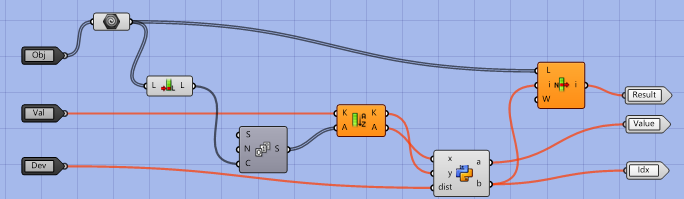# Group points in the list based on x coordinate value range

Hi, I have a list of points
I want to group points in the list based on x coordinate value
and the maximum difference in x value for each group will be fix number ( ex .1500)

Welcome @tarek2721978,

It’s customary here to upload a file with externalised example data, if you require help.
More on the dos and don’ts of this forum can be found here:

``````from Grasshopper.Kernel.Data import GH_Path
from Grasshopper import DataTree

dataTree1 = DataTree[object]()
dataTree2 = DataTree[object]()
j = 0
for i in range(len(y)):

if abs(y[i] - y[i-1]) <= dist and i != 0:
pass
else:
j += 1
branch_id = j - 1

path = GH_Path(branch_id)